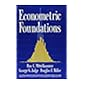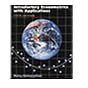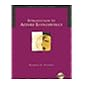Normal view MARC view ISBD view

# Econometric foundations / Ron C. Mittelhammer, George G. Judge, Douglas J. Miller.

Material type:TextPublisher: New York : Cambridge University Press, 2000Description: xxviii, 756 p. : ill. ; 26 cm. +.ISBN: 0521623944 hb; 9780521623940 hb.DDC classification: 330.015195 Online resources: WorldCat details
Contents:
Table of contents The process of econometric information recovery -- Probability-econometric models -- The multivariate normal linear regression model: ML estimation -- The multivariate normal linear regression model: inference -- The linear semiparametric regression model: least-squares estimation -- The linear semiparametric regression model: inference -- Extremum estimators and nonlinear and nonnormal regression models -- The nonlinear semiparametric regression model: estimation and inference -- Nonlinear and nonnormal parametric regression models -- Stochastic regressors and moment-based estimation -- Quasi-maximum likelihood and estimating equations -- Empirical likelihood estimation and inference -- Information theoretic-entropy approaches to estimation and inference -- Regression models with a known general noise covariance matrix -- Regression models with an unknown general noise covariance matrix -- Generalized moment-based estimation and inference -- Simultaneous equations econometric models: estimation and inference -- Model discovery: the problem of variable selection and conditioning -- Model discovery: the problem of noise covariance matrix specification -- Qualitative-censored response models -- Introduction to nonparametric density and regression analysis -- Bayesian estimation: general principles with a regression focus -- Alternative Bayes formulations for the regression model -- Bayesian inference -- Appendix: Introduction to computer simulation and resampling methods.
Summary: Summary: This textbook and accompanying CD-ROM develop step by step a modern approach to econometric problems.
Tags from this library: No tags from this library for this title.Average rating: 0.0 (0 votes)
Item type Current location Collection Call number Copy number Status Date due Barcode Item holdsText
Reserve Section
Non-fiction 330.015195 MIE 2000 (Browse shelf) C-1 Not For Loan 27331CDs & DVDs
Audio Visual
Non-fiction 330.015195 MIE 2000 (Browse shelf) C-1 Available CD-1509
Total holds: 0
##### Browsing EWU Library Shelves , Shelving location: Audio Visual Close shelf browser330.015195 GUB 2003 Basic econometrics / 330.015195 GUB 2003 Basic econometrics / 330.015195 GUB 2003 Basic econometrics / 330.015195 MIE 2000 Econometric foundations / 330.015195 RAI 2002 Introductory econometrics with applications / 330.015195 STI 2005 Introduction to applied econometerics / 330.015195 WOE 2009 Econometrics /

Includes bibliographical references and index.

Probability-econometric models --
The multivariate normal linear regression model: ML estimation --
The multivariate normal linear regression model: inference --
The linear semiparametric regression model: least-squares estimation --
The linear semiparametric regression model: inference --
Extremum estimators and nonlinear and nonnormal regression models --
The nonlinear semiparametric regression model: estimation and inference --
Nonlinear and nonnormal parametric regression models --
Stochastic regressors and moment-based estimation --
Quasi-maximum likelihood and estimating equations --
Empirical likelihood estimation and inference --
Information theoretic-entropy approaches to estimation and inference --
Regression models with a known general noise covariance matrix --
Regression models with an unknown general noise covariance matrix --
Generalized moment-based estimation and inference --
Simultaneous equations econometric models: estimation and inference --
Model discovery: the problem of variable selection and conditioning --
Model discovery: the problem of noise covariance matrix specification --
Qualitative-censored response models --
Introduction to nonparametric density and regression analysis --
Bayesian estimation: general principles with a regression focus --
Alternative Bayes formulations for the regression model --
Bayesian inference --
Appendix: Introduction to computer simulation and resampling methods.

Summary:
This textbook and accompanying CD-ROM develop step by step a modern approach to econometric problems.

Economics

There are no comments for this item.